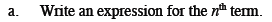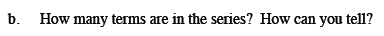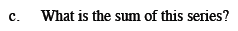Home > CCA2 > Chapter Ch10 > Lesson 10.1.2 > Problem10-35

10-35.
1. Consider the series 21 + 17 + 13 + … + −99 as you answer the questions in parts (a) through (c) below. Homework Help ✎

1. Write an expression for the nth term.

2. How many terms are in the series? How can you tell?

3. What is the sum of this series?Use a system of equations or look at the difference between terms and the value of the first term.Set the equation you found in part (a) equal to −99 and solve for n.Use the rectangle method or Antonio's method.

−1209